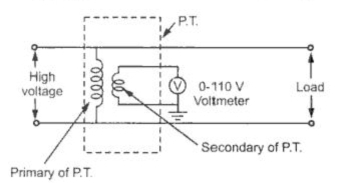PotentialTransformer (P.T.)

The basic principle of these transformers is same as current transformers. The high alternating voltage are reduced in a fixed proportion for the measurement purpose with the help of potential transformers. Ths construction of these transformers is similar to the normal transformer. These are extremely accurate ratio step down transformers. The windings are low power rating windings. Primary winding consists of large number of turns while secondary has less number of turns and usually rated for 110 V, irrespective of the primary voltage rating. The primary is connected across the high voltage line while secondary is connected to the low range voltmeter coil. One end of the secondary is always grounded for safety purpose. The connections are shown in the Fig. 1.Fig. 1  Potential transformer

As a normal transformer, its ratio can be specified as,
V1/V2 = N1/N2
So if voltage ratio of P.T. is known and the voltmeter reading is known the the high voltage to be measured, can be determined.
Example :  A 11000 : 110, potential transformer is used along with a voltmeter reading 87.5 V. Estimate the value of line voltage.
Solution : For a P.T.                       V1/V2 = 11000/110
and                                                  V2 = 87.5 V
...                                                     V1/87.5 = 11000/110
...                                                      V1 = 8750 V
This is the value of high voltage to be measured.
1.1 Construction
The potential transformer use large core and conductor sizes compared to conventional power transformer. In potential transformer, economy of material is not a important consideration at the time of design. The accuracy is an important consideration.
The shell type or core type construction is preferred for potential transformer. The shell type is used for low voltage while core type for high voltage transformers. At the time of assembly special core is required to reduce the effect of air gap at the joints.
The coaxial primary and secondary windings are used, to reduce the leakage reactance. ye secondary winding which is a low voltage winding is always next to the core. The primary winding is a single coil in low voltage transformers. For high voltages, insulation is the main problem. Hence in high voltage potential transformers, primary is divided into number of small sections of short coils to reduce the need of insulation between coil layers.
The cotton type and varnished cambric are used as the insulations for windings. Hard fiber separators are used in between the coils. The oil immersed potential transformers are used for the voltages levels above 7 kV. For oil filled potential transformers, oil filled bushings are used. Two bushings are required when no side of the line is at earth potential.
The overall construction of single phase, two windings potential transformer is known in the Fig. 2.Fig. 2  Single phase potential transformer
Related Articles :